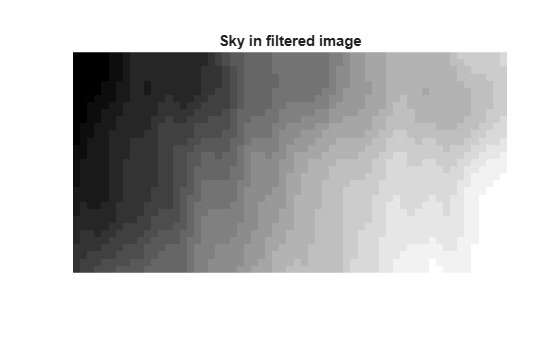Apply Gaussian Smoothing Filters to Images

This example shows how to apply different Gaussian smoothing filters to images using imgaussfilt. Gaussian smoothing filters are commonly used to reduce noise.

Read an image into the workspace.

Filter the image with isotropic Gaussian smoothing kernels of increasing standard deviations. Gaussian filters are generally isotropic, that is, they have the same standard deviation along both dimensions. An image can be filtered by an isotropic Gaussian filter by specifying a scalar value for sigma.

Iblur1 = imgaussfilt(I,2);
Iblur2 = imgaussfilt(I,4);
Iblur3 = imgaussfilt(I,8);

Display the original image and all the filtered images.

figure
imshow(I)
title('Original image')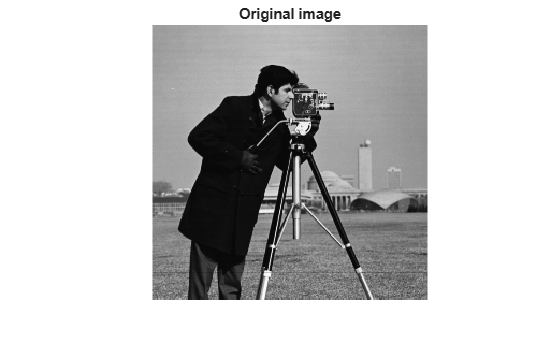figure
imshow(Iblur1)
title('Smoothed image, \sigma = 2')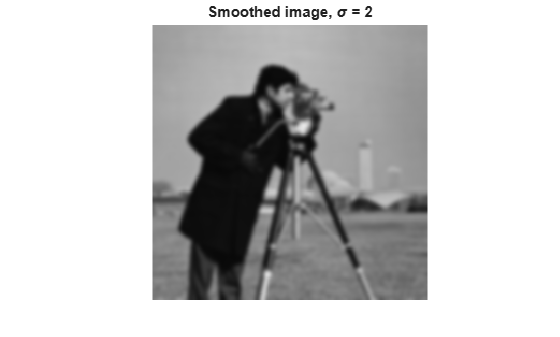figure
imshow(Iblur2)
title('Smoothed image, \sigma = 4')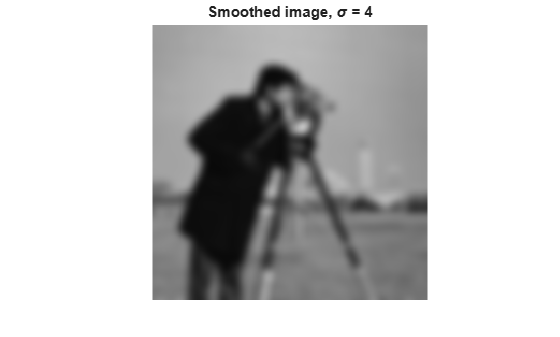figure
imshow(Iblur3)
title('Smoothed image, \sigma = 8')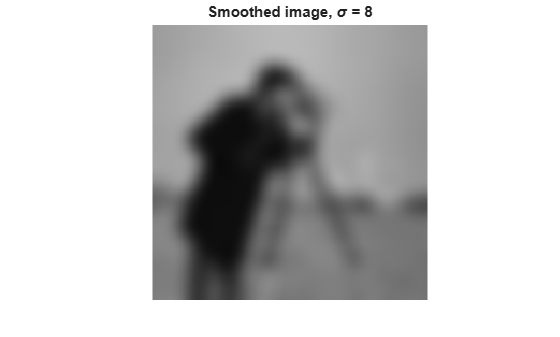Filter the image with anisotropic Gaussian smoothing kernels. imgaussfilt allows the Gaussian kernel to have different standard deviations along row and column dimensions. These are called axis-aligned anisotropic Gaussian filters. Specify a 2-element vector for sigma when using anisotropic filters.

IblurX1 = imgaussfilt(I,[4 1]);
IblurX2 = imgaussfilt(I,[8 1]);
IblurY1 = imgaussfilt(I,[1 4]);
IblurY2 = imgaussfilt(I,[1 8]);

Display the filtered images.

figure
imshow(IblurX1)
title('Smoothed image, \sigma_x = 4, \sigma_y = 1')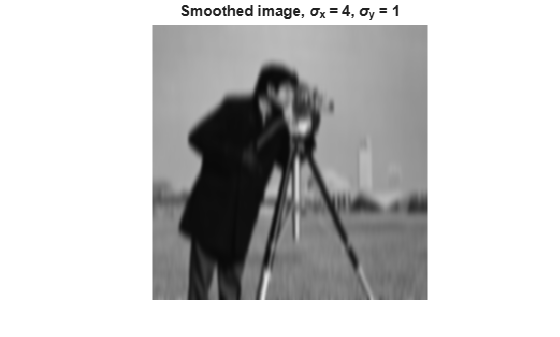figure
imshow(IblurX2)
title('Smoothed image, \sigma_x = 8, \sigma_y = 1')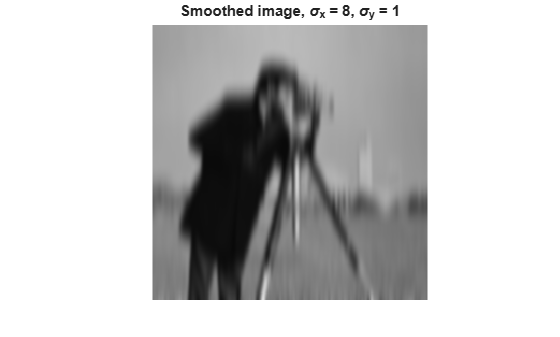figure
imshow(IblurY1)
title('Smoothed image, \sigma_x = 1, \sigma_y = 4')figure
imshow(IblurY2)
title('Smoothed image, \sigma_x = 1, \sigma_y = 8')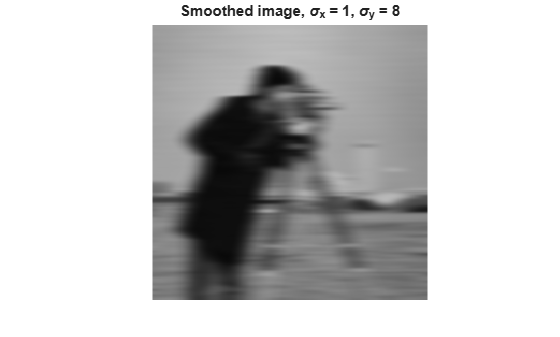Suppress the horizontal bands visible in the sky region of the original image. Anisotropic Gaussian filters can suppress horizontal or vertical features in an image. Extract a section of the sky region of the image and use a Gaussian filter with higher standard deviation along the X axis (direction of increasing columns).

Display the original patch of sky with the filtered version.

figure
imshow(I_sky), title('Sky in original image')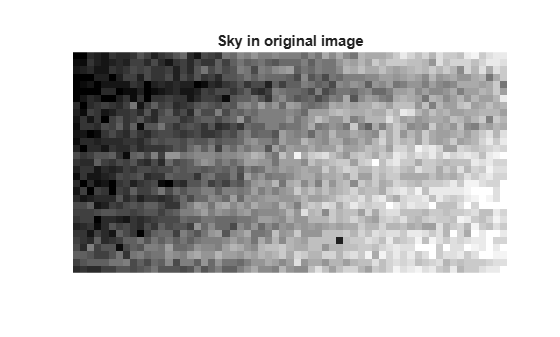figure
imshow(IblurX1_sky), title('Sky in filtered image')